## ML Aggarwal Class 7 Solutions for ICSE Maths Chapter 10 Lines and Angles Objective Type Questions

Mental Maths

Question 1.
Fill in the blanks:
(i) If two angles are complementary, then the sum of their measures is ………..
(ii) If two angles are supplementary, then the sum of their measures is …………
(iii) Supplement of an obtuse angle is ……….
(iv) Two angles forming a linear pair are ……….
(v) If two adjacent angles are supplementary, then they form a ………..
(vi) Angles of a linear pair are as well as ………..
(vii) Adjacent angles have a common vertex, a common ……….. and no common ………
(viii)Angles formed by two intersecting lines having no common arms are called ………..
(ix) If two lines intersect and if one pair of vertically opposite angles are acute angles, then the other pair of vertically opposite angles are ……..
(x) Two lines in a plane which never meet are called ……….
(xi) Alternate interior angles have one common …………
(xii) Corresponding angles are on the ……….. side of the transversal.
(xiii) Alternate interior angles are on the ………… side of the transversal.
(xiv) If two lines are cut by a transversal such that a pair of corresponding angles are not equal, then the lines are ………..
Solution:
(i) If two angles are complementary,
then the sum of their measures is 90°.
(ii) If two angles are supplementary,
then the sum of their measures is 180°.
(iii) Supplement of an obtuse angle is an acute angle.
(iv) Two angles forming a linear pair are supplementary.
(v) If two adjacent angles are supplementary,
then they form a linear pair.
(vi) Angles of a linear pair are adjacent as well as supplementary.
(vii) Adjacent angles have a common vertex,
a common arm, and no common interior points.
(viii) Angles formed by two intersecting lines having
no common arms are called vertically opposite angles.
(ix) If two lines intersect and if one pair of vertically opposite angles
are acute angles, then the other pair of vertically opposite angles are obtuse angles.
(x) Two lines in a plane which never meet are called parallel lines.
(xi) Alternate interior angles have one common arm.
(xii) Corresponding angles are the same side of the transversal.
(xiii) Alternate interior angles are on the opposite side of the transversal.
(xiv) If two lines are cut by a transversal such that a pair of
corresponding angles are not equal, then the lines are not parallel.

Question 2.
In the given figure, AB is a straight line and OD ⊥ AB. Observe the figure and fill in the following blanks:(i) ∠AOC and ∠COE form a pair of ……….. angles.
(ii) ∠AOC and ∠COB are ……….. angles.
(iii) ∠AOC is ………… of ∠COD.
(iv) ∠BOE is …………. of ∠EOA.
Solution:
In the given figure,
AB is a straight line and OD ⊥ AB
(i) ∠AOC and ∠COE form a pair of adjacent angles.
(ii) ∠AOC and ∠COB are supplementary angles.
(iii) ∠AOC is the complement of ∠COD.
(iv) ∠BOE is a supplement of ∠EOA.

Question 3.
State whether the following statements are true (T) or false (F):
(i) Two obtuse angles can be supplementary.
(ii) Two acute angles can form a linear pair.
(iii) Two obtuse angles can form a linear pair.
(iv) Two adjacent angles always form a linear pair.
(v) Pair of vertically opposite angles are always supplementary.
(vi) 30° is one-half of its complement.
(vii) If two lines are cut by a transversal,
then each pair of corresponding angles are equal.
(viii) If two lines are cut by a transversal,
then each pair of alternate interior angles are equal.
Solution:
(i) Two obtuse angles can be supplementary. (False)
Correct:
As the sum of two obtuse angles is greater than 180°.
(ii) Two acute angles can form a linear pair. (False)
Correct:
As the sum of two acute angles is less than 180°.
(iii) Two obtuse angles can form a linear pair. (False)
Correct :
As the sum of two obtuse angles is greater than 180°.
(iv) Two adjacent angles always form a linear pair. (False)
Correct:
As the sum of two adjacent angles can be less than 180° or more than 180°.
(v) Pair of vertically opposite angles are always supplementary. (False)
(vi) 30° is one-half of its complement. (True)
(30° and 60° are complement and 30° = $$\frac { 1 }{ 2 }$$ × 60°)
(vii) If two lines are cut by a transversal,
then each pair of corresponding angles are equal. (False)
Correct:
It may or may not be equal.
If lines are parallel than the corresponding angle are the same
and if lines are not parallel than angles are different.
(viii) If two lines are cut by a transversal,
then each pair of alternate interior angles are equal. (False)
Correct :
If the two lines are parallel, then it is possible.
Otherwise, these angles are different.

Multiple Choice Questions

Choose the correct answer from the given four options (4 to 14):
Question 4.
A pair of complementary angles is
(a) 130°, 50°
(b) 35°, 55°
(c) 25°, 75°
(d) 27°, 53°
Solution:
A pair of complementary angles is 35°, 55°.
As the sum of complementary angles is 90°. (b)

Question 5.
A pair of supplementary angles is
(a) 55°, 115°
(b) 65°, 125°
(b) 47°, 133°
(d) 40°, 50°
Solution:
A pair of supplementary angles is 47°, 133°.
As the sum of supplementary angles is 180°. (c)

Question 6.
If an angle is one-third of its supplement, then the measure of the angle is
(a) 45°
(b) 30°
(c) 135°
(d) 150°
Solution:
If one angle is one-third of its supplement,
then the measure of an angle is 45°. (a)
Let first angle = x°x = $$\frac { 180\times 3 }{ 4 }$$ = 135°

Question 7.
If an angle measures 10° more than its complement, then the measure of the angle is
(a) 40°
(b) 55°
(c) 35°
(d) 50°
Solution:
If one angle measures 10° more than its complement,
then measure of the angle = 50°.
(∵ 50° + 40° = 90°)

Question 8.
If one angle of a linear pair is acute, then the other angle is
(a) acute
(b) obtuse
(c) right
(d) straight
Solution:
If one angle of a linear pair is acute,
then the other angle will be obtuse.
(∵ Linear pair = 180°) (b)

Question 9.
In the given figure, the value of x that will make AOB a straight line is
(a) x = 40
(b) x = 35
(c) x = 30
(d) x = 25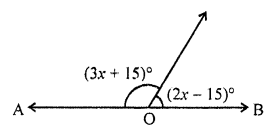Solution:
AOB will be a straight line if
3x + 15 + 2x – 10° = 180°
⇒ 5x + 5 = 180°
⇒ 5x = 180° – 5 = 175°
⇒ x = 35° (b)

Question 10.
If two lines are intersected by a transversal, then the number of pairs of interior angles on the same side of transversal is
(a) 1
(b) 2
(c) 3
(d) 4
Solution:
Two lines are intersected by a transversal then a number of
pairs of interior angles on the same side of the transversal is two. (b)

Question 11.
In the given figure, if l || m then the value of x is
(a) x = 50
(b) x = 60
(c) x = 70
(d) x = 45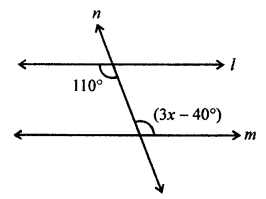Solution:
In the given figure, l || m
110° = 3x – 40°
⇒ 3x = 110° + 40° = 150°
x = 50° (a)

Question 12.
In the gitsen figure, if l || m then the value of x is
(a) x = 75°
(b) x = 95°
(c) x = 105°
(d) x = 115°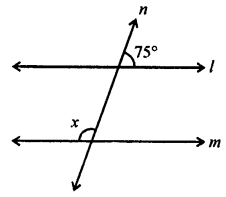Solution:
In the given figure, l || m
∠1 = 75° (Vertically opposite angles)
and ∠1 + x = 180° (Co-interior angles)
x + 75° = 180°
x = 180° – 75° = 105° (c)Question 13.
In the given figure, AB || CD. If ∠APQ = 50° and ∠PRD = 130°, then ∠QPR is
(a) 30°
(b) 50°
(c) 80°
(d) 130°Solution:
In the given figure,
AB || CD, ∠APQ = 50°
∠PRD = 130°, ∠QPR = ?
AB || CD
∠APQ = ∠PQR (Alternate angles)
∠PQR = 50°
∠PRD + ∠PRQ = 180° (Linear pair)
130° + ∠PRQ = 180°
∠PRQ = 180° – 130° = 50°
Now in ∆PQR
∠PQR + ∠PRQ + ∠QPR = 180° (Angles of a triangle)
50° + 50° + ∠QPR = 180°
∠QPR = 180° – 50° – 50° = 80° (c)

Question 14.
In the given figure, PA || BC || DQ and AB || DC. Then the values of x and y are respectively:
(a) 50°, 120°
(b) 50°, 130°
(c) 60°, 120°
(d) 60°, 130°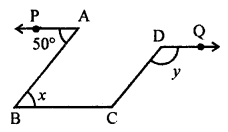Solution:
In the given figure,
PA || BC || DQ
AB || DC
x = 50° (Alternate angles)
∠BCD + ∠ABC = 180° (Sum of co-interior angles)
⇒ ∠DCB + 50° = 180°
⇒ ∠DCB = 180° – 50° = 130°
BC || DQ
∠DCB = ∠CDQ (Alternate angles)
y = 130°
x = 50°, y = 130° (b)

Higher Order Thinking Skills (HOTS)

Question 1.
Calculate the measure of each lettered angle in the following figures (parallel line segments / rays are denoted by thick matching arrows):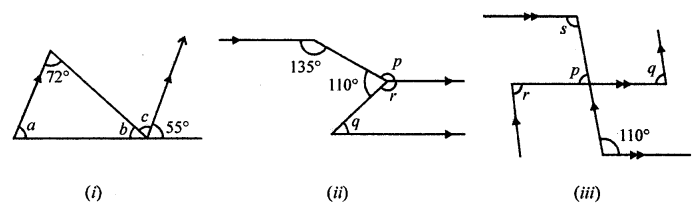Solution:
(i) In the given figure,
Given ∠s are 72° and 55°
a = 55° (Corresponsing angles)
c = 72° (Alternate angles)
But b + c + 55° = 180°
(Angles on the one side of a straight line)
⇒ b + 72° + 55° = 180°
⇒ b + 127° = 180°
⇒ b = 180° – 127° = 53°
a = 55, b = 53°, c = 72°
(ii) In the given figure,Given ∠s are 135° and 110°
p = 135° (Alternate angles)
p + r + 110° = 360° (Angles at a point)
⇒ 135° + 110° + r = 360°
⇒ 245° + r = 360°
⇒ r = 360° – 245° = 115°
But r + q = 180° (Co-interior angles)
⇒ 115° + q = 180°
⇒ q = 180° – 115° = 65°
p = 135°, q = 65°, r = 115°
(iii) In the given figure,
Given angle is 110°
p = ∠1 (Vertically opposite angles)
But ∠1 + 110° = 180° (Co-interior angles)
⇒ ∠1 = 180° – 110° = 70°
⇒ p = ∠1 = 70°
s = 110° (Alternate angles with 110°)
p = q (Corresponding angles)
q = 70°
r = q (Alternate angles)
r = 70°
Now, p = 70°, q = 70°, r = 70°, s = 110°